# Question:why Latex removes constant from expression when it is at front?

## Question:why Latex removes constant from expression when it is at front?

Maple 2020

I am using solution given in How-To-Make-Sort-Work-With-Alias which works well.

But on more testing, I found that Latex drops the constant of integration after applying the above solution, when this constant shows at the front of the rhs of the expression.

I have no idea why this happens. I will post two examples. The first shows the problem with the Latex generated, showing the constant is missing from the Latex. The second example is where I moved the constant  to inside the expression keeping everything else the same, and now the Latex generated no longer drops it.

This is serious problem, since now my solutions are losing constant of integration in Latex display depending on where it is located.

example 1

restart;
assign(seq(print/_C||k, k=0..10) = seq(c[k], k=0..10)):
S:=[seq(_C||k = _C||k(), k=0..10)]:

sol:= y(x) = _C1/cosh(x)+(cosh(x)^2+cosh(x)*sinh(x)+x)/cosh(x);
sol:=subs(S, sort(sol));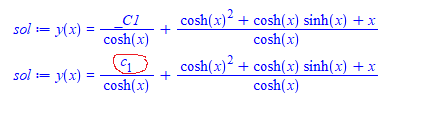Now watch what happens to the Latex of the above

Latex(sol)

y \! \left(x \right) =
\frac{1}{\cosh \! \left(x \right)}+\frac{\cosh^{2}x +\cosh \! \left(x \right) \sinh \! \left(x \right)+x}{\cosh \! \left(x \right)}


Which compiles to

\documentclass[11pt]{article}
\usepackage{amsmath}
\begin{document}
$y \! \left(x \right) = \frac{1}{\cosh \! \left(x \right)}+\frac{\cosh^{2}x +\cosh \! \left(x \right) \sinh \! \left(x \right)+x}{\cosh \! \left(x \right)}$
\end{document}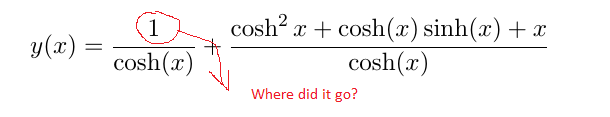Now will do the same, but move the constant to the second term. Now it shows up

example 2

restart;
assign(seq(print/_C||k, k=0..10) = seq(c[k], k=0..10)):
S:=[seq(_C||k = _C||k(), k=0..10)]:

sol:= y(x) = 1/cosh(x)+ _C1*(cosh(x)^2+cosh(x)*sinh(x)+x)/cosh(x);
sol:=subs(S, sort(sol));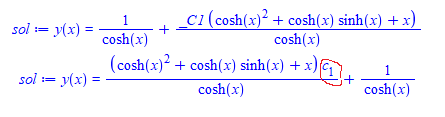Now generate the latex

Latex(sol)

y \! \left(x \right) =
\frac{\left(\cosh^{2}x +\cosh \! \left(x \right) \sinh \! \left(x \right)+x \right) c_{1}}{\cosh \! \left(x \right)}+\frac{1}{\cosh \! \left(x \right)}



Which compiles to

\documentclass[11pt]{article}
\usepackage{amsmath}
\begin{document}
$y \! \left(x \right) = \frac{\left(\cosh^{2}x +\cosh \! \left(x \right) \sinh \! \left(x \right)+x \right) c_{1}}{\cosh \! \left(x \right)}+\frac{1}{\cosh \! \left(x \right)}$
\end{document}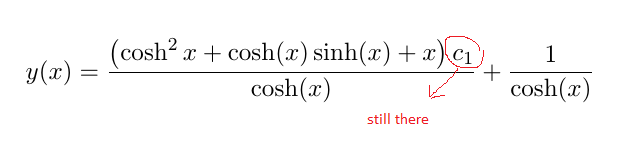I am using 2020.2 with Physics 893 on windows 10.

Any idea what is going on? And why this happens when constant is at front only?

ps. the Purpose of using the solution in the above link, is to be able to use c instead of _C1 in the final latex, and also have these constants show at front (this is what sort does) which makes the final expression better looking).

Thank you

Edit

In the debugger, going over Latex() function, I found where the problem happen.  There is a call near line 20 which does this

Latex:-Print(e,':-executeprintroutines');

Where is the input. Which in this case is the sol expression passed to the Latex command.

Removing ':-executeprintroutines' from the above call, the constant remain there and is not lost.

It seems assign(seq(print/_C||k, k=0..10) = seq(c[k], k=0..10)): used before the call to Latex is confusing things.

Here is illustration:

restart;
assign(seq(print/_C||k, k=0..10) = seq(c[k], k=0..10)):
S:=[seq(_C||k = _C||k(), k=0..10)]:
sol:= y(x) = _C1/cosh(x)+(cosh(x)^2+cosh(x)*sinh(x)+x)/cosh(x);
sol:=subs(S, sort(sol));

Latex:-Print(sol,':-executeprintroutines');
Latex:-Print(sol);


And the output of the last 2 commands is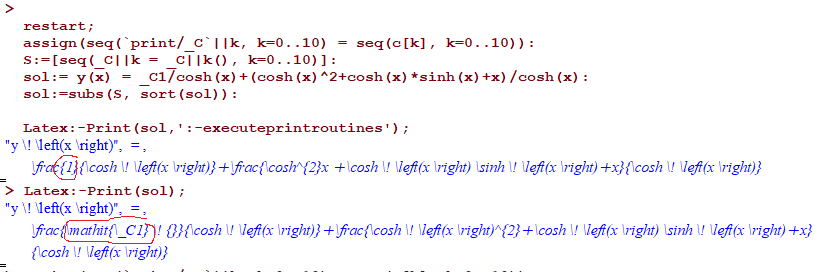This shows when using ':-executeprintroutines' the constant is lost. I do not know what ':-executeprintroutines' does or where it is defined to try to modify it.﻿